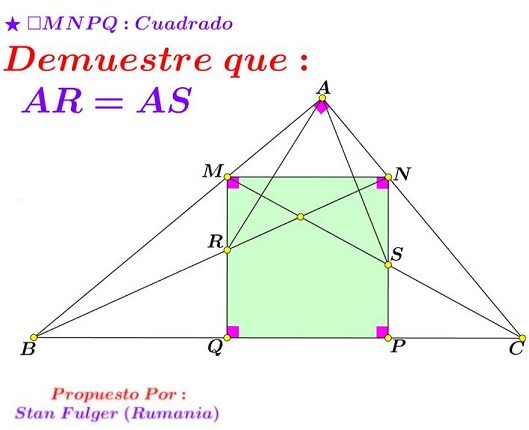## Stan Fulger's Observation in Right Triangle

### Source### Problem### Solution

First off, let's find the side of the square in terms of the side lengths $a,b,c\,$ of the triangle.

If $x=MN=MQ,\,$ $h\,$ the altitude in $\Delta ABC\,$ from $A,\,$ then using the similarity of triangles $ABC\,$ and $AMN,\,$ $\displaystyle\frac{h-x}{h}=\frac{x}{a},\,$ wherefrom $x=\displaystyle\frac{ha}{h+a}.\,$Recollecting that the area of a right triangle can be computed in two ways, we get $bc=ha,\,$ of $\displaystyle h=\frac{bc}{a}.\,$ Combining the two expressions gives

$\displaystyle x=\frac{abc}{a^2+bc}.$

It follows that $\displaystyle AM=\frac{bc^2}{bc+a^2}\,$ and $\displaystyle AM=\frac{b^2c}{bc+a^2}.\,$ Now then, from the similarity of triangles $AMN\,$ and $QBM,\,$ $BQ=\displaystyle\frac{ac^2)(bc+a^2}\,$ and, similarly, $\displaystyle CP=\frac{ab^2}{bc+a^2}.$

From the construction, there's a square, $JUQR\,$ with $J\,$ on $AB\,$ and $U\,$ on $BC.\,$ We can again use similarity of triangles or remark that $QJ\,$ is an angle bisector in $\Delta BQM\,$ and find its length with a well-known formula. Thus, we can find the side of the square $\displaystyle QR=\frac{ab^2c}{(bc+a^2)(b+c)}.\,$ Similarly, $\displaystyle PS=\frac{abc^2}{(bc+a^2)(b+c)}.\,$ Note that $QR+PS=x,\,$ implying $PS=MR\,$ and $QR=NS\,$ such that $RS\,$ go0es through the center $X\,$ of the square $MNPQ.\,$ In particular, $RX=SX.$

If now $O\,$ is the midpoint of $UV,\,$ then,

1. $OP:OQ=b:c=QR:PS\,$ and
2. $BO:CO=(BQ+PS):(CP+QR)=c:b.$

It follows that $AO\,$ bisects angle $BAC\,$ and, denoting its intersection with $MN\,$ as $O',\,$ $MO':NO'=c:b\,$ so that $OO'\,$ passes through $X\,$ and is perpendicular to $RS.\,$ In $\Delta RAS,\,$ $AX\,$ serves as the median and the altitude from $A\,$ which makes the triangle $isosceles:\,$ $AR=AS.$

### Acknowledgment

This problem by Stan Fulger has been posted at the Peru Geometrico facebook group by Miguel Ochoa Sanchez.